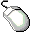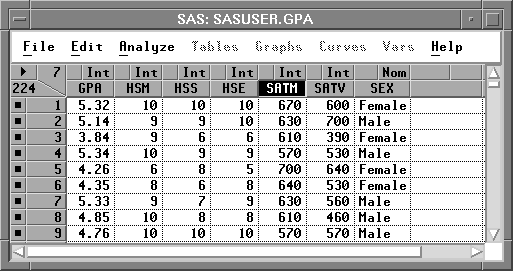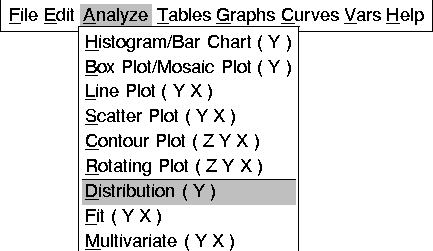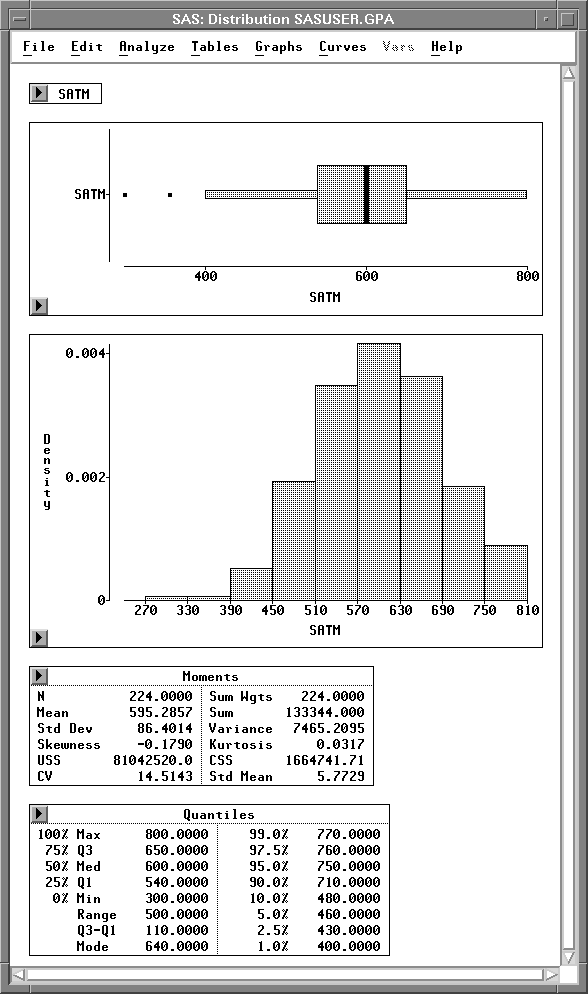Examining Distributions

# Creating the Distribution Analysis

The distribution of a variable is the pattern of variation of its numerical values (Moore and McCabe 1989). In this example, you examine a distribution of scores on the mathematics portion of the SAT exam.Open the GPA data set.Select the variable SATM by clicking on its name in the data window.Figure 12.2: Data Window with SATM SelectedChoose Analyze:Distribution ( Y ).This creates a distribution window, as shown in Figure 12.4. A box plot, histogram, Moments table, and Quantiles table appear by default. With these graphs and tables, you can examine important features of a distribution.Figure 12.4: Distribution Analysis